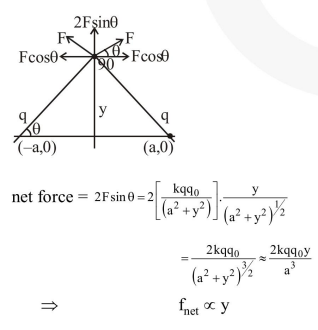# Two charges each equal toq$are kept at x=-a and x=a on the x-axis.Question: Two charges each equal to$\mathrm{q}$are kept at$\mathrm{x}=-\mathrm{a}$and$\mathrm{x}=\mathrm{a}$on the$\mathrm{x}$-axis. A particle of mass$\mathrm{m}$and charge$\mathrm{q}_{0}=\frac{\mathrm{q}}{2}$is placed at the origin. If charge$\mathrm{q}_{0}$is given a small displacement$(\mathrm{y}<<\mathrm{a})$along the$\mathrm{y}$-axis, the net force acting on the particle is proportional to 1. y 2. - y 3.$\frac{1}{y}$4.$-\frac{1}{y}\$

Correct Option: 1

Solution: